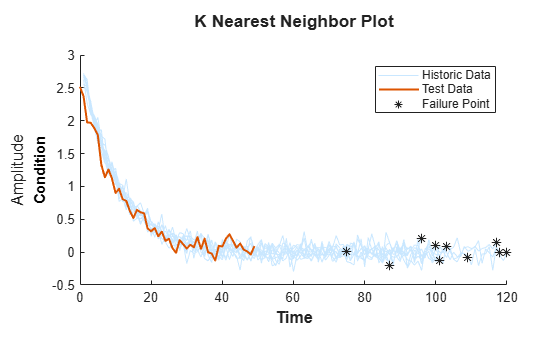# compare

Compare test data to historical data ensemble for similarity models

## Syntax

``compare(mdl,data)``
``compare(___,Name,Value)``

## Description

example

````compare(mdl,data)` plots the test component degradation data in `data` superimposed on the most similar data sets from the historical ensemble stored in the fitted similarity model `mdl`. The K most similar data sets from the ensemble are plotted, where K is the `NumNearestNeighbors` property of `mdl`.```

example

````compare(___,Name,Value)` specifies plotting options using one or more name-value pair arguments.```

## Examples

collapse all

`load('pairwiseTrainTables.mat')`

The training data is a cell array of tables. Each table is a degradation feature profile for a component.

Create and train a pairwise similarity model.

```mdl = pairwiseSimilarityModel; fit(mdl,pairwiseTrainTables,"Time","Condition")```

`load('pairwiseTestData.mat')`

Compare the degradation profile of the test data to the profiles of the historical data ensemble.

`compare(mdl,pairwiseTestData)``load('pairwiseTrainTables.mat')`

The training data is a cell array of tables. Each table is a degradation feature profile for a component.

Create and train a pairwise similarity model.

```mdl = pairwiseSimilarityModel; fit(mdl,pairwiseTrainTables,"Time","Condition")```

`load('pairwiseTestData.mat')`

Compare the degradation profile of the test data to the profiles of the `10` most similar members of the historical data ensemble.

`compare(mdl,pairwiseTestData,'NumNearestNeighbors',10)`## Input Arguments

collapse all

Similarity RUL model, specified as a `hashSimilarityModel` object, a `pairwiseSimilarityModel` object, or a `residualSimilarityModel` object. The model must be fitted using `fit` before calling `compare`.

Degradation feature profiles for estimating the RUL of similarity models, measured over the life span of a component up to the current life time, specified as one of the following:

• N-by-(Mi+1) arrays, where N is the number of feature measurements (at different usage times) and Mi is the number of features. The first column contains the usage times and the remaining columns contain the corresponding measurements for degradation features. The order of the features must match the order specified in the `DataVariables` property of ` mdl`.

• `table` or `timetable` object — The table must contain variables with names that match the strings in the `DataVariables` and `LifeTimeVariable` properties of `mdl`.

### Name-Value Arguments

Specify optional pairs of arguments as `Name1=Value1,...,NameN=ValueN`, where `Name` is the argument name and `Value` is the corresponding value. Name-value arguments must appear after other arguments, but the order of the pairs does not matter.

Before R2021a, use commas to separate each name and value, and enclose `Name` in quotes.

Example: `'NumNearestNeighbors','10'` plots ten similar data sets

Number of nearest neighbors, specified as the comma-separated pair `'NumNearestNeighbors'` and either `Inf` or a finite positive integer. Use this option to select the number of most similar data sets to plot by overriding the `NumNearestNeighbors` property. If `NumNearestNeighbors` is `Inf`, then `compare` plots the degradation data for all the ensemble data sets.

Degradation data bounds, specified as the comma-separated pair `'Threshold'` and a two-column array with N rows, where N is the number of data variables used by `mdl`. The first column of `Threshold` contains the lower bounds for the variables, and the second column contains the upper bounds. The bounds are rendered as yellow-colored patches.

To disable the bounds for a given variable, specify the lower and upper bounds as `-Inf` and `Inf`, respectively.

## Tips

• To select which signals to plot, right-click on the plot area, and select Data Variable Selector. In the Data Variable Selector dialog box, the Select Variables box shows the variables that are available for plotting.

## Version History

Introduced in R2018a